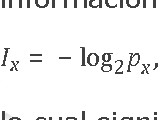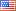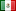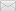# Information theory

## with intuitive examples on personal data

### by Alejandro Ochoa García

Names, birthdates, addresses, and other data.VIIIA- -## Introduction

Have you ever wondered how useful it is when a random company online collects your name, address, maybe your birthdate, and sometimes much more? What makes that information useful? Can we quantify it?

This is an article for people that like math, but it can be recreationally and doesn't go beyond basic college level math. If you remember how logarithms work, you're set, but if not I try to go slow anyway. Probability here usually reduces to simple fractions, so advance knowledge of that field isn't necessary either, except that independent probabilities can be multiplied. Only once I use a Bernoulli distribution, which you can safely ignore.

Lastly, the equations on this page are written using MathML, which works out of the box with Firefox but not with most other browsers, although a MathML plugin is available for IE (MathPlayer). I think you have no choice if you use Safari or Chrome except to wait until those browsers support it. This will probably make little sense without the equations.

## Theory

Shannon, the inventor of modern information theory, uses this definition as the the basis of information,

${I}_{x}=-{\mathrm{log}}_{2}{p}_{x},$

meaning that the information of an event x is greater as the probability of such an event gets smaller. In other words, an event has more information if it is harder to guess. But that's not all. The information of the union of two independent events is the sum of their individual informations. Explicitly in equations,

${p}_{\mathrm{x,y}}={p}_{x}\cdot {p}_{y}$
${I}_{\mathrm{x,y}}=-{\mathrm{log}}_{2}{p}_{\mathrm{x,y}}=-{\mathrm{log}}_{2}\left({p}_{x}\cdot {p}_{y}\right)=-{\mathrm{log}}_{2}{p}_{x}-{\mathrm{log}}_{2}{p}_{y}={I}_{x}+{I}_{y}\text{.}$

This is one of the reasons the logarithm is a natural choice to map probabilities into information, since only the logarithm (of any base) can map arbitrary products into sums.

Often you don't have an exact value for your random variable X, but you'd like to know how informative X is on average. It turns out this quantity is used all the time in probability, and it's called the Entropy of X (or H(X)).

$H\left(X\right)=E\left[{I}_{X}\right]={\sum }_{x}{p}_{x}\cdot {I}_{x}=-{\sum }_{x}{p}_{x}{\mathrm{log}}_{2}{p}_{x}.$

## Human population as a probability space

It's easy to talk about information about people. In this theory, we need a probability space into which we can score information. The easiest experiment involves considering each person as an entity in the probability space, and every property (or random variable) X acquires a probability by adding the number of people that have such a property and divide it over the human population.

According to the U.S. Census Bureau, the world population is projected to be 7 billion by April, 2012 (accessed on 2011-09-04). That means that the maximum information possible in this setting, enough to narrow down to a single person, corresponds to

${I}_{x}=-{\mathrm{log}}_{2}\frac{1}{n}={\mathrm{log}}_{2}n={\mathrm{log}}_{2}7,000,000,000=32.7\text{bits}.$

Of course, the minimum ammount of information in this and any other setting is 0 bits, here corresponding to any property that all humans have (p=1). For example, if I told you I have a heart, that would carry zero information in narrowing down which human I am.

Information as defined here also corresponds to the minimum amount of storage needed to identify events in this space. So it seems incredible to me that you could identify each human currently alive uniquely using only 33 bits (or a little over 4 bytes). Using the estimate of Carl Haub, the number of people that have ever lived on Earth totals 106 billion people, so the number of bits needed to identify any of them is

${I}_{x}={\mathrm{log}}_{2}106,456,367,669=36.63\text{bits}.$

## Gender: 1 bit

The human population is almost exactly evenly male and female,

${p}_{\text{male}}={p}_{\text{female}}=\frac{1}{2}\text{,}$

so knowing my gender is worth

${I}_{x}=-{\mathrm{log}}_{2}\frac{1}{2}=1\text{bit.}$

Althought it seems small, gender is very often independent of other kinds of information (for example, geography, but not names), so you can easily and reliably add 1 bit of information by asking for the gender.

## Geography (address): highly informative

Using published statistics about the population of these different regions (often approximations from Wikipedia), knowing where I live in increasing resolution affords you with the following information.

${I}_{\text{USA}}=-{\mathrm{log}}_{2}\frac{312,138,791}{7,000,000,000}=4.49\text{bits.}$
${I}_{\text{NJ}}=-{\mathrm{log}}_{2}\frac{8,791,894}{7,000,000,000}=9.64\text{bits.}$
${I}_{\text{ZIP 08540}}=-{\mathrm{log}}_{2}\frac{56,534}{7,000,000,000}=16.92\text{bits.}$
${I}_{\text{Princeton}}=-{\mathrm{log}}_{2}\frac{30,000}{7,000,000,000}=17.83\text{bits.}$

I was surprised the city affords more information than the zip code in this case, but I'm sure it varies depending on how big your city is (if it has multiple zip codes).

I was a bit disturbed by the detailed stats on my zip code available on city-data.com. For example, if you knew I'm a hispanic living in 08540, you'd have

${I}_{\text{ZIP 08540 & Hispanic}}=-{\mathrm{log}}_{2}\frac{1,757}{7,000,000,000}=21.93\text{bits.}$

If you know where I work or study, you get a similar amount of information.

${I}_{\text{Princeton University student}}=-{\mathrm{log}}_{2}\frac{7,567}{7,000,000,000}=19.82\text{bits.}$
${I}_{\text{Princeton University graduate student}}=-{\mathrm{log}}_{2}\frac{2,479}{7,000,000,000}=21.43\text{bits.}$

I don't know how informative a street address is. My street is very short, it has 16 buildings, each with 3 units, each of which usually houses at least 2 people, but sometimes a bit more. If we settle for an average of 3 people per unit, we'd have

${I}_{\text{my street}}=-{\mathrm{log}}_{2}\frac{16\cdot 3\cdot 3}{7,000,000,000}=25.53\text{bits.}$

And if you have my unit number, you actually have nearly the maximum information,

${I}_{\text{my street & unit number}}=-{\mathrm{log}}_{2}\frac{3}{7,000,000,000}=31.12\text{bits,}$

since currently that information narrows things down to me, my wife and my baby. However, the reality is a bit less ideal. Addresses expire regularly, especially on a college town. I regularly get mail addressed to former inhabitants, so many of these businesses certainly have old information. My address has been used to identify up to maybe 7 couples or roommates in the last 10 years, which might reduce the information to

${I}_{\text{my street & unit number, corrected}}=-{\mathrm{log}}_{2}\frac{3\cdot 7\cdot 2}{7,000,000,000}=27.31\text{bits.}$

## Names: > 12 bits

Limited to the USA, you can find out how common your first and last names are on HowManyOfMe.com. Although the website suggests it knows how many people with both your first and last name are, further reading shows that they assume independence, and they acknowledge that this is an incorrect assumption. We'll therefore ignore this information.

I got the following statistics for my name

${I}_{\text{Alejandro}}=-{\mathrm{log}}_{2}\frac{67,092}{312,138,791}=12.18\text{bits.}$
${I}_{\text{Ochoa}}=-{\mathrm{log}}_{2}\frac{66,179}{312,138,791}=12.20\text{bits.}$
${I}_{\text{García}}=-{\mathrm{log}}_{2}\frac{992,848}{312,138,791}=8.30\text{bits.}$

Note I included my second family name, a feature of names in Spanish-speaking countries. I imagine in those cases that the two family names are combined randomly (meaning people don't generally choose who they marry by their family name). In that case, the information content of a double famly name is, on average, twice that of a single family name, as it is used in the USA. However, this still doesn't guarantee that the name will uniquely identify me. In fact, I've known of a person matching my full Spanish name in my hometown of Juárez, and maybe less surprisingly, his brother matched my brother's full name as well.

The same website suggests that James and Smith are independently the most common first and last names in the USA. The numbers for these least informative names look like this.

${I}_{\text{James}}=-{\mathrm{log}}_{2}\frac{5,192,584}{312,138,791}=5.91\text{bits.}$
${I}_{\text{Smith}}=-{\mathrm{log}}_{2}\frac{2,748,738}{312,138,791}=6.83\text{bits.}$

Similarly, Muhammad and Zhang are reported to be respectively the most common first and last names in the world. Their information content is

${I}_{\text{Muhammad}}=-{\mathrm{log}}_{2}\frac{150,000,000}{7,000,000,000}=5.54\text{bits.}$
${I}_{\text{Zhang}}=-{\mathrm{log}}_{2}\frac{100,000,000}{7,000,000,000}=6.13\text{bits.}$

So adding these minumum information contents, and rounding up for a bit of optimism, we see that the minimum information content of a full name is about 12 bits. So although some names are greatly informative (I believe my son has a unique name, a combination of a Scottish, an English, a Basque, and a Dutch name), it is interesting to see that there is a relatively high minimum information in a name. This makes sense, otherwise names would be useless, not really serving their purpose of identifying people.

Note that first names are highly correlated with gender (an Alejandro is all but certainly male). The map is of course complicated, so many websites will ask for both pieces of data anyway, but their information is usually not additive. Similarly, names and geography are highly correlated (my name is a lot more informative in the USA than it would be in Mexico). On the other hand, names are overall very likely to be independent from birthdates (next), so we can add their information.

I did want to mention interesting exceptions of the independence between names and birthdates. One I remember is from Mexico, where people used to be named (at least one of your first or middle names) after the saint on whose feast day they were born. At least I think that's how some of my aunts were named, but Wikipedia would only confirm this tradition for Italy. In a different example, Ghanaian names are usually chosen from the day of the week a person was born. In either example, the information of a name and a birthdate is smaller than the sum of the independent informations, but we can easily remove the components that are dependent (usually a single name) to get a more decent approximation.

## Birthdates: ~ 14 bits

How much information is in a birthday (day and month, but not year for now)? To get a quick answer, let's oversimplify the problem. Assume there are exactly 365 days in a year and that being born on any day is equally likely. In that case a birthday gives us this much information.

${I}_{\text{birth day and month}}={\mathrm{log}}_{2}365=8.51\text{bits.}$

Of course, things are known to be more complicated. Roy Murphy has found that birthdays are significantly non-uniform. He specifically found that births are slightly more likely in July through October, while they are less likely March through May. David Gleich has also found a significant bias in births by weekday, in a subset of Americans, with fewer births during the weekend, which might be explained by C-sections and inductions being more common during the week.

In the end, a less uniform birthday distribution implies that the average information content of a birthday is less that what we've estimated. This is because the entropy of a random variable is maximal when its distrubution is uniform.

If you add a birth year, this is additional information equivalent to knowing your age. Assuming you're alive, the range of ages is for the most part limited to 0 through 85 years. If you again assumed a uniform distribution over that range, you'd find that this age/year adds this much information.

${I}_{\text{birth year}}={\mathrm{log}}_{2}86=6.43\text{bits.}$

So combining the birth day and month with the year information, which are most likely independent, you get a full 14.94 bits with a full birthdate.

The age distribution is known to be highly skewed towards the younger ages. You can see the exact distribution for Americans. So again the implication is that the actual age information is lower than we've calculated it to be. Younger ages are less informative, while older ages are more informative.

## Number of legs: very uninformative

I wanted to give a detailed example of when something is very uninformative. The vast majority of people have 2 legs, but a small fraction only have 1 or even 0, having lost them in accidents or for other reasons. I imagine there are people with more than two legs, due to genetic or developmental anomalies, but to simplify this analysis I'll ignore those cases. I looked online for a leg distribution, and couldn't find one. So I'll have to invent data for my example.

Assume the probability of losing a leg is

${p}_{\text{lost leg}}=\frac{1}{10,000},$

and the loss of each leg is an independent event, so the distribution of number of lost legs is binomial with n=2. In that case each of the events has this much information.

${I}_{\text{2 legs}}={\mathrm{log}}_{2}{\left(1-\frac{1}{10,000}\right)}^{2}=0.000289\text{bits.}$
${I}_{\text{1 leg}}={\mathrm{log}}_{2}\left(2\cdot \frac{1}{10,000}\left(1-\frac{1}{10,000}\right)\right)=12.29\text{bits.}$
${I}_{\text{0 legs}}={\mathrm{log}}_{2}{\left(\frac{1}{10,000}\right)}^{2}=26.58\text{bits.}$

Interestingly, knowing that somebody has less than 2 legs can be extremely informative. So why don't online forms ask for your number of legs more often? The secret is in the average information content, or entropy, of the number of legs. The problem with the very informative cases is that they are very rare, so when you weigh their information by their probability, their effect is reduced relative to the majority of low-information 2-leg cases. In equations,

$H\left(\text{legs}\right)=-{p}_{\text{2 legs}}{\mathrm{log}}_{2}{p}_{\text{2 legs}}-{p}_{\text{1 leg}}{\mathrm{log}}_{2}{p}_{\text{1 leg}}-{p}_{\text{0 legs}}{\mathrm{log}}_{2}{p}_{\text{0 legs}}=0.002746\text{bits.}$

In general, we'll have a low average information (or low entropy) when one or very few cases dominate. This is why you're not normally asked online how many legs you have.

## Eye color: ~ 1.5 bits in USA

I tried looking for data on eye color, but this is the best I could do, found on Yahoo answers, and except for "American Academy of Opthamology", I could not find the source or other information on the following data.

${I}_{\text{blue/grey irises}}={\mathrm{log}}_{2}\frac{32}{100}=1.64\text{bits.}$
${I}_{\text{blue/grey/green irises with brown/yellow specks}}={\mathrm{log}}_{2}\frac{15}{100}=2.74\text{bits.}$
${I}_{\text{green/light brown irises with minimal specks}}={\mathrm{log}}_{2}\frac{12}{100}=3.06\text{bits.}$
${I}_{\text{brown irises with specks}}={\mathrm{log}}_{2}\frac{16}{100}=2.64\text{bits.}$
${I}_{\text{dark brown irises}}={\mathrm{log}}_{2}\frac{25}{100}=2\text{bits.}$
$H\left(\text{eye color}\right)=1.54\text{bits.}$

The situation is much worse worldwide, with brown eyes dominating over the rest, and consequently the entropy drops to nearly zero (same as in the "number of legs" example).

Although slightly more informative than gender in USA (and independent of it), eye color is not independent of geography and names, which are by themselves much more informative, so it might make sense that nobody asks for your eye color in webforms.

## Conclusions

We could estimate that name and birthdate information are independent and highly informative, combined providing at least 26 bits of information, sometimes much more depending on the name. Geography, or street address, is also highly informative, and while not independent of the previous quantities, combined most likely narrows the vast majority of people.

I hope this exercise has helped you understand in intuitive terms how information theory works, and maybe you can apply it to your data of interest.